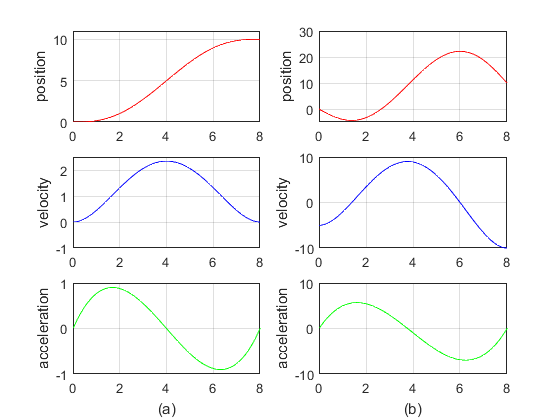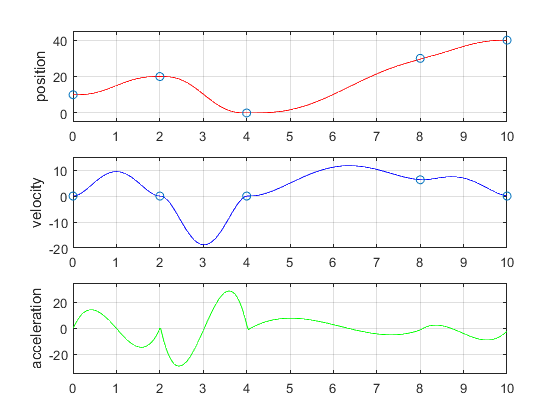• 多项式定义多项式是代数学中的基础概念，是由称为不定元的变量和称为系数的常数通过有限加减法、乘法以及自然数幂的乘方运算得到的代数表达式。例如X2 - 3X + 4就是多项式多项式是整式的种。不定元...

多项式函数以其简单的结构和性质在数值逼近中起到重要的作用,多项式的定义是什么?以下是学习啦小编为大家整理的关于多项式的定义，欢迎大家前来阅读!
多项式的定义
多项式是代数学中的基础概念，是由称为不定元的变量和称为系数的常数通过有限次加减法、乘法以及自然数幂次的乘方运算得到的代数表达式。例如X2 - 3X + 4就是一个多项式。多项式是整式的一种。不定元只有一个的多项式称为一元多项式;不定元不止一个的多项式称为多元多项式。多项式在数学的很多分支中乃至许多自然科学以及工程学中都有重要作用。
多项式数学术语
多项式 polynomial
不含字母的项叫做常数项。如：5X+6，6就是常数项。
比较广义的定义，1个或0个单项式的和也算多项式。按这个定义，多项式就是整式。实际上，还没有一个只对狭义多项式起作用，对单项式不起作用的定理。0作为多项式时，次数为正无穷大。单项式和多项式统称为整式。
多项式几何特性
多项式是简单的连续函数，它是平滑的，它的微分也必定是多项式。
泰勒多项式的精神便在于以多项式逼近一个平滑函数，此外闭区间上的连续函数都可以写成多项式的均匀极限。
多项式定理
基本定理
代数基本定理是指所有一元 n 次(复数)多项式都有 n 个(复数)根。
高斯引理
两个本原多项式的乘积是本原多项式。
应用高斯引理可证，如果一个整系数多项式可以分解为两个次数较低的有理系数多项式的乘积，那么它一定可以分解为两个整系数多项式的乘积。这个结论可用来判断有理系数多项式的不可约性。关于Q[x]中多项式的不可约性的判断，还有艾森斯坦判别法：对于整系数多项式，如果有一个素数p能整除αn-1，αn-2，…，α1，α0，但不能整除αn，且p2不能整除常数项α0，那么ƒ(x)在Q上是不可约的。由此可知，对于任一自然数n，在有理数域上xn-2是不可约的。因而，对任一自然数n，都有n次不可约的有理系数多项式。
分解定理
F[x]中任一个次数不小于 1的多项式都可以分解为F上的不可约多项式的乘积，而且除去因式的次序以及常数因子外，分解的方法是惟一的。
当F是复数域C时，根据代数基本定理，可证C[x]中不可约多项式都是一次的。因此，每个复系数多项式都可分解成一次因式的连乘积。
当F是实数域R时，由于实系数多项式的虚根是成对出现的，即虚根的共轭数仍是根，因此R[x]中不可约多项式是一次的或二次的。所以每个实系数多项式都可以分解成一些一次和二次的不可约多项式的乘积。实系数二次多项式αx2+bx+с不可约的充分必要条件是其判别式b2-4αс<0。
当F是有理数域Q时，情况复杂得多。要判断一个有理系数多项式是否不可约，就较困难。应用本原多项式理论，可把有理系数多项式的分解问题化为整系数多项式的分解问题。一个整系数多项式如其系数是互素的，则称之为本原多项式。每个有理系数多项式都可表成一个有理数及一个本原多项式的乘积。关于本原多项式有下述重要性质。
多项式运算法则
加法与乘法
有限个单项式之和称为多元多项式，简称多项式。不同类的单项式之和表示的多项式，其中系数不为零的单项式的最高次数，称为此多项式的次数。
多项式的加法，是指多项式中同类项的系数相加，字母保持不变(即合并同类项)。多项式的乘法，是指把一个多项式中的每个单项式与另一个多项式中的每个单项式相乘之后合并同类项。
F上x1，x2，…，xn的多项式全体所成的集合F[x1，x2，…，xn]，对于多项式的加法和乘法成为一个环，是具有单位元素的整环。
域上的多元多项式也有因式分解惟一性定理。
带余除法
若 ƒ(x)和g(x)是F[x]中的两个多项式，且 g(x)≠0，则在F[x]中有唯一的多项式 q(x)和r(x)，满足ƒ(x)=q(x)g(x)+r(x)，其中r(x)的次数小于g(x)的次数。此时q(x) 称为g(x)除ƒ(x)的商式，r(x)称为余式。当g(x)=x-α时，则r(x)=ƒ(α)称为余元，式中的α是F的元素。此时带余除法具有形式ƒ(x)=q(x)(x-α)+ƒ(α)，称为余元定理。g(x)是ƒ(x)的因式的充分必要条件是g(x)除ƒ(x)所得余式等于零。如果g(x)是ƒ(x)的因式，那么也称g(x) 能整除ƒ(x)，或ƒ(x)能被g(x)整除。特别地，x-α是ƒ(x)的因式的充分必要条件是ƒ(α)=0，这时称α是ƒ(x)的一个根。
如果d(x)既是ƒ(x)的因式，又是g(x)的因式，那么称d(x)是ƒ(x)与g(x)的一个公因式。如果d(x)是ƒ(x)与g(x)的一个公因式，并且ƒ(x)与g(x)的任一个因式都是d(x)的因式，那么称d(x)是ƒ(x)与g(x)的一个最大公因式。如果ƒ(x)=0，那么g(x)就是ƒ(x)与g(x)的一个最大公因式。当ƒ(x)与g(x)全不为零时，可以应用辗转相除法来求它们的最大公因式。
辗转相除法
已知一元多项式环F[x] 中两个不等于零的多项式ƒ(x)与g(x)，用g(x)除ƒ(x)得商式q1(x)、余式r1(x)。若r1(x)=0，则g(x)就是ƒ(x)与g(x)的一个最大公因式。若 r1(x)≠0，则用 r1(x)除 g(x)得商式q2(x)、余式r2(x)。若r2(x)=0，则r1就是ƒ(x)与g(x)的一个最大公因式。否则，如此辗转相除下去，余式的次数不断降低，经有限s次之后，必有余式为零次(即零次多项式)或余式为零(即零多项式)。若最终余式结果为零次多项式，则原来f(x)与g(x)互素;若最终余式结果为零多项式，则原来f(x)与g(x)的最大公因式是最后一次带余除法的是除式。
利用辗转相除法的算法，可将ƒ(x)与g(x)的最大公因式rs(x)表成ƒ(x)和g(x)的组合，而组合的系数是F上的多项式。
如果ƒ(x)与g(x)的最大公因式是零次多项式，那么称ƒ(x)与g(x)是互素的。最大公因式和互素概念都可以推广到几个多项式的情形。
如果F[x]中的一个次数不小于1的多项式ƒ(x)，不能表成 F[x] 中的两个次数较低的多项式的乘积，那么称ƒ(x)是F上的一个不可约多项式。
任一多项式都可分解为不可约多项式的乘积。
多项式应用
函数及根
给出多项式 f∈R[x1，...，xn] 以及一个 R-代数 A。对 (a1...an)∈An，我们把 f 中的 xj 都换成 aj，得出一个 A 中的元素，记作 f(a1...an)。如此， f 可看作一个由 An 到 A 的函数。
若然 f(a1...an)=0，则 (a1...an) 称作 f 的根或零点。
例如 f=x^2+1。若然考虑 x 是实数、复数、或矩阵，则 f 会无根、有两个根、及有无限个根!
例如 f=x-y。若然考虑 x 是实数或复数，则 f 的零点集是所有 (x，x) 的集合，是一个代数曲线。事实上所有代数曲线由此而来。
另外，若所有系数为实数多项式 P(x)有复数根Z，则Z的共轨复数也是根。
若P(x)有n个重叠的根，则 P‘(x) 有n-1个重叠根。即若 P(x)=(x-a)^nQ(x)，则有 a 是 P’(x)的重叠根且有n-1个。
插值多项式
在实际问题中，往往通过实验或观测得出表示某种规律的数量关系y=F(x)，通常只给出了F(x)在某些点xi上的函数值yi=F(xi)，j=1，2，…，n+1。即使有时给出了函数F(x)的解析表达式，倘若较为复杂，也不便于计算。因此，需要根据给定点 xi 上的函数值F(xi)，求出一个既能反映F(x)的特性，又便于计算的简单函数ƒ(x)来近似地代替F(x)，此时ƒ(x)称为F(x)的插值函数;x1，x2，…，xn+1，称为插值节点。求插值函数的方法，称为插值法。
多项式是一类简单的初等函数，而且任给两组数：b1，b2，…，bn+1和各不相同的 с1，с2，…，сn+1，总有唯一的次数不超过n的多项式ƒ(x)满足ƒ(сi)=bi，i=1，2，…，n+1。因此在实际应用中常常取多项式作为插值函数。作为插值函数的多项式，称为插值多项式。插值多项式在计算数学插值中最常用。
看过"多项式的定义"的人还喜欢看：

展开全文• [duō xiàng shì]多项式语音编辑锁定讨论上传视频在数学中，由若干个单项式相加组成的代数式叫做...中文名多项式外文名polynomial适用领域应用学科数学定义连续函数多项式定义编辑语音在数学中，多项式(polynom...

[duō xiàng shì]
多项式
语音
编辑
锁定
讨论
上传视频
在数学中，由若干个单项式相加组成的代数式叫做多项式(若有减法：减一个数等于加上它的相反数)。多项式中的每个单项式叫做多项式的项，这些单项式中的最高项次数，就是这个多项式的次数。其中多项式中不含字母的项叫做常数项。
中文名
多项式
外文名
polynomial适用领域
应用学科
数学
定    义
连续函数
多项式定义
编辑
语音
在数学中，多项式(polynomial)是指由变量、系数以及它们之间的加、减、乘、幂运算(非负整数次方)得到的表达式。
对于比较广义的定义，1个或0个单项式的和也算多项式。按这个定义，多项式就是整式。实际上，还没有一个只对狭义多项式起作用，对单项式不起作用的定理。0作为多项式时，次数定义为负无穷大(或0)。单项式和多项式统称为整式。
多项式中不含字母的项叫做常数项。如：5X+6中的6就是常数项。
多项式几何特性
编辑
语音
多项式是简单的连续函数，它是平滑的，它的微分也必定是多项式。
泰勒多项式的精髓便在于以多项式逼近一个平滑函数，此外闭区间上的连续函数都可以写成多项式的均匀极限。
多项式定理
编辑
语音
多项式基本定理
代数基本定理是指所有一元 n 次(复数)多项式都有 n 个(复数)根。
多项式高斯引理
两个本原多项式的乘积是本原多项式。
应用高斯引理可证，如果一个整系数多项式可以分解为两个次数较低的有理系数多项式的乘积，那么它一定可以分解为两个整系数多项式的乘积。这个结论可用来判断有理系数多项式的不可约性。关于Q[x]中多项式的不可约性的判断，还有艾森斯坦判别法：对于整系数多项式,如果有一个素数p能整除αn-1,αn-2,…,α1,α0，但不能整除αn,且pˆ2不能整除常数项α0，那么ƒ(x)在Q上是不可约的。由此可知，对于任一自然数n，在有理数域上xn-2是不可约的。因而，对任一自然数n，都有n次不可约的有理系数多项式。
多项式分解定理
F[x]中任一个次数不小于 1的多项式都可以分解为F上的不可约多项式的乘积，而且除去因式的次序以及常数因子外，分解的方法是惟一的。
当F是复数域C时，根据代数基本定理，可证C[x]中不可约多项式都是一次的。因此，每个复系数多项式都可分解成一次因式的连乘积。
当F是实数域R时，由于实系数多项式的虚根是成对出现的，即虚根的共轭数仍是根，因此R[x]中不可约多项式是一次的或二次的。所以每个实系数多项式都可以分解成一些一次和二次的不可约多项式的乘积。实系数二次多项式αx2+bx+с不可约的充分必要条件是其判别式b2-4αс<0。
当F是有理数域Q时，情况复杂得多。要判断一个有理系数多项式是否不可约，就较困难。应用本原多项式理论，可把有理系数多项式的分解问题化为整系数多项式的分解问题。一个整系数多项式如其系数是互素的,则称之为本原多项式。每个有理系数多项式都可表成一个有理数及一个本原多项式的乘积。关于本原多项式有下述重要性质。
多项式运算法则
编辑
语音
多项式加法与乘法
有限的单项式之和称为多项式。不同类的单项式之和表示的多项式，其中系数不为零的单项式的最高次数，称为此多项式的次数。
多项式的加法，是指多项式中同类项的系数相加，字母保持不变(即合并同类项)。多项式的乘法,是指把一个多项式中的每个单项式与另一个多项式中的每个单项式相乘之后合并同类项。
F上x1，x2，…，xn的多项式全体所成的集合Fx{1,x2，…,xn}，对于多项式的加法和乘法成为一个环，是具有单位元素的整环。
域上的多元多项式也有因式分解惟一性定理。
多项式带余除法
若 f(x)和g(x)是F[x]中的两个多项式，且g(x)不等于0，则在F[x]中有唯一的多项式 q(x)和r(x)，满足ƒ(x)=q(x)g(x)+r(x)，其中r(x)的次数小于g(x)的次数。此时q(x) 称为g(x)除ƒ(x)的商式，r(x)称为余式。当g(x)=x-α时，则r(x)=ƒ(α)称为余元，式中的α是F的元素。此时带余除法具有形式ƒ(x)=q(x)(x-α)+ƒ(α)，称为余元定理。g(x)是ƒ(x)的因式的充分必要条件是g(x)除ƒ(x)所得余式等于零。如果g(x)是ƒ(x)的因式，那么也称g(x) 能整除ƒ(x)，或ƒ(x)能被g(x)整除。特别地，x-α是ƒ(x)的因式的充分必要条件是ƒ(α)=0，这时称α是ƒ(x)的一个根。
如果d(x)既是ƒ(x)的因式，又是g(x)的因式，那么称d(x)是ƒ(x)与g(x)的一个公因式。如果d(x)是ƒ(x)与g(x)的一个公因式，并且ƒ(x)与g(x)的任一个因式都是d(x)的因式，那么称d(x)是ƒ(x)与g(x)的一个最大公因式。如果ƒ(x)=0，那么g(x)就是ƒ(x)与g(x)的一个最大公因式。当ƒ(x)与g(x)全不为零时，可以应用辗转相除法来求它们的最大公因式。
多项式辗转相除法
已知一元多项式环F[x]中两个不等于零的多项式ƒ(x)与g(x)，用g(x)除ƒ(x)得商式q1(x)、余式r1(x)。若r1(x)=0，则g(x)就是ƒ(x)与g(x)的一个最大公因式。若 r1(x)≠0，则用 r1(x)除 g(x)得商式q2(x)、余式r2(x)。若r2(x)=0,则r1就是ƒ(x)与g(x)的一个最大公因式。否则，如此辗转相除下去,余式的次数不断降低，经有限s次之后，必有余式为零次(即零次多项式)或余式为零(即零多项式)。若最终余式结果为零次多项式，则原来f(x)与g(x)互素；若最终余式结果为零多项式，则原来f(x)与g(x)的最大公因式是最后一次带余除法的是除式。
利用辗转相除法的算法，可将ƒ(x)与g(x)的最大公因式rs(x)表成ƒ(x)和g(x)的组合，而组合的系数是F上的多项式。
如果ƒ(x)与g(x)的最大公因式是零次多项式，那么称ƒ(x)与g(x)是互素的。最大公因式和互素概念都可以推广到几个多项式的情形。
如果F[x]中的一个次数不小于1的多项式ƒ(x)，不能表成 F[x] 中的两个次数较低的多项式的乘积，那么称ƒ(x)是F上的一个不可约多项式。
任一多项式都可分解为不可约多项式的乘积。
形如 Pn(x)=a(n)x^n+a(n-1)x^(n-1)+…+a(1)x+a(0)的函数，叫做函数，它是由常数与自变量x经过有限次乘法与加法运算得到的。显然，当n=1时，其为一次函数y=kx+b，当n=2时，其为二次函数y=ax^2+bx+c。
多项式应用
编辑
语音
多项式函数及其根
给出多项式 f∈R[x1,...,xn] 以及一个 R-代数 A。对 (a1，...，an)∈An，我们把 f 中的 xj都换成 aj，得出一个 A 中的元素，记作 f(a1...an)。如此， f 可看作一个由 An 到 A 的函数。
若然 f(a1...an)=0，则 (a1...an) 称作 f 的根或零点。
例如 f=x^2+1。若然考虑 x 是实数、复数、或矩阵，则 f 会无根、有两个根、及有无限个根！
例如 f=x-y。若然考虑 x 是实数或复数，则 f 的零点集是所有 (x,x) 的集合，是一个代数曲线。事实上所有代数曲线由此而来。
另外，若所有系数为实数多项式 P(x)有复数根Z，则Z的共轨复数也是根。
若P(x)有n个重叠的根，则 P‘(x) 有n-1个重叠根。即若 P(x)=(x-a)^nQ(x)，则有 a 是 P’(x)的重叠根且有n-1个。
多项式插值多项式
在实际问题中，往往通过实验或观测得出表示某种规律的数量关系y=F(x)，通常只给出了F(x)在某些点xi上的函数值yi=F(xi)，j=1，2，…,n+1。即使有时给出了函数F(x)的解析表达式，倘若较为复杂，也不便于计算。因此，需要根据给定点 xi 上的函数值F(xi),求出一个既能反映F(x)的特性，又便于计算的简单函数ƒ(x)来近似地代替F(x),此时ƒ(x)称为F(x)的插值函数；x1,x2,…,xn+1，称为插值节点。求插值函数的方法，称为插值法。
多项式是一类简单的初等函数，而且任给两组数：b1,b2,…,bn+1和各不相同的 с1,с2,…,сn+1，总有唯一的次数不超过n的多项式ƒ(x)满足ƒ(сi)=bi，i=1，2，…,n+1。因此在实际应用中常常取多项式作为插值函数。作为插值函数的多项式，称为插值多项式。插值多项式在计算数学插值中最常用。
词条图册
更多图册
参考资料
1.
王东明，牟晨琪，李晓亮 等著．多项式代数：高等教育出版社，2011
2.
王萼芳等．高等代数：高等教育出版社，2003年

展开全文• 利用三次多项式，根据过q0,q1,…,qnq_0,q_1,\ldots,q_nq0​,q1​,…,qn​确定的轨迹的特征是位置和速度连续，但是加速度不连续（参见上篇博客轨迹规划–三次多项式轨迹）。尽管三次多项式轨迹确定的轨迹有一定...
#多项式轨迹–五次多项式
1.5 Polynomial of degree five
利用三次多项式，根据过

q

0

,

q

1

,

…

,

q

n

q_0,q_1,\ldots,q_n

确定的轨迹的特征是位置和速度连续，但是加速度不连续（参见上一篇博客轨迹规划–三次多项式轨迹）。尽管三次多项式轨迹确定的轨迹有一定“平滑”，但是对于一些应用的动力学和惯性载荷会产生一些不期待的影响。为了获得一个加速度连续的轨迹，位置和速度需要合适的初始和终止条件，也需要合适的初始和终止加速度值。这样共有六个边界条件，因此需要采用五次多项式：

q

(

t

)

=

q

0

+

a

1

(

t

−

t

0

)

+

a

2

(

t

−

t

0

)

2

+

a

3

(

t

−

t

0

)

3

+

a

4

(

t

−

t

0

)

4

+

a

5

(

t

−

t

0

)

5

(1-24)

q(t)=q_0+a_1(t-t_0)+a_2(t-t_0)^2+a_3(t-t_0)^3+a_4(t-t_0)^4+a_5(t-t_0)^5 \tag{1-24}

根据条件

q

(

t

0

)

=

q

0

,

q

(

t

1

)

=

q

1

q

˙

(

t

0

)

=

v

0

,

q

˙

(

t

1

)

=

v

1

q

¨

(

t

0

)

=

a

0

,

q

¨

(

t

1

)

=

a

1

.

\begin{matrix} q(t_0)=q_0, &q(t_1)=q_1 \\ \dot{q}(t_0)=v_0, & \dot{q}(t_1)=v_1 \\ \ddot{q}(t_0)=\text a_0, & \ddot{q}(t_1)=\text a_1. \end{matrix}

这里定义

T

=

t

1

−

t

0

T=t_1-t_0

,求得多项式系数为

{

a

0

=

a

0

a

1

=

v

0

a

2

=

1

2

a

0

a

3

=

1

2

T

3

[

20

h

−

(

8

v

1

+

12

v

0

)

T

−

(

3

a

0

−

a

1

)

T

2

]

a

4

=

1

2

T

4

[

−

30

h

+

(

14

v

1

+

16

v

0

)

T

+

(

3

a

0

−

2

a

1

)

T

2

]

a

5

=

1

2

T

5

[

12

h

−

6

(

v

1

+

v

0

)

T

+

(

a

1

−

a

0

)

T

2

]

.

(1-25)

\begin{cases} a_0&=&a_0\\ a_1&=&v_0\\ a_2&=&\frac{1}{2}\text a_0\\ a_3&=&\frac{1}{2T^3}\left[20h-\left(8v_1+12v_0\right)T-(3\text a_0-\text a_1)T^2\right]\\ a_4&=&\frac{1}{2T^4}[-30h+(14v_1+16v_0)T+(3\text a_0-2\text a_1)T^2]\\ a_5&=&\frac{1}{2T^5}[12h-6(v_1+v_0)T+(\text a_1-\text a_0)T^2]. \end{cases} \tag{1-25}

Example2.9:使用五次多项式确定运动轨迹：
(a)

t

0

=

0

,

t

1

=

8

,

q

0

=

0

,

q

1

=

10

,

v

0

=

0

,

v

1

=

0

,

a

0

=

0

,

a

1

=

0.

\begin{matrix} t_0=0,& t_1=8, \\ q_0=0,& q_1=10, \\ v_0=0, &v_1=0,\\ \text a_0=0, &\text a_1=0. \end{matrix}

(b) $$\begin{matrix} t_0&=&0,& t_1&=&8, \ q_0&=&0,& q_1&=&10, \ v_0&=&-5, &v_1&=&-10,\ \text a_0&=&0, & \text a_1&=&0. \end{matrix}$$ 可以注意到，使用三次多项式是无法指定加速度的边界条件的。对(a)和(b)条件使用五次多项式确定轨迹如下图所示。可以与上一篇博文的三次多项式（轨迹规划–三次多项式轨迹）比较一下，三次多项式是无法指定加速度的边界条件的。图7 五次多项式轨迹
matlab仿真代码参见examplesCode文件夹下的example2_9.m文件
%example2.9
clc
clear
%轨迹定义条件
%时间
t0=0;
t1=8;
%位置和速度（a）
q0=0;
q1=10;
v0=0;
v1=0;
acc0=0;
acc1=0;
%利用公式（1-25）求系数
h=q1-q0;
T=t1-t0;
a0=q0;
a1=v0;
a2=1.0/2*acc0;
a3=1.0/(2*T*T*T)*(20*h-(8*v1+12*v0)*T+(acc1-3*acc0)/(T*T));
a4=1.0/(2*T*T*T*T)*(-30*h+(14*v1+16*v0)*T+(3*acc0-2*acc1)/(T*T));
a5=1.0/(2*T*T*T*T*T)*(12*h-6*(v1+v0)*T+(acc1-acc0)/(T*T));
%轨迹生成
t=t0:0.1:t1;
%位置
q=a0+a1*power((t-t0),1)+a2*power((t-t0),2)+a3*power((t-t0),3)+...
a4*power(t-t0,4)+a5*power(t-t0,5);
%速度
v=a1+2*a2*power((t-t0),1)+3*a3*power((t-t0),2)+4*a4*power(t-t0,3)+...
5*a5*power(t-t0,4);
%加速度
acc=2*a2+6*a3*power((t-t0),1)+12*a4*power(t-t0,2)+20*a5*power(t-t0,3);
%绘图
subplot(3,2,1)
plot(t,q,'r');
axis([0,8,0,11])
ylabel('position')
grid on
subplot(3,2,3)
plot(t,v,'b');
axis([0,8,-1,2.5])
ylabel('velocity')
grid on
subplot(3,2,5)
plot(t,acc,'g');
xlabel('(a)');
ylabel('acceleration')
grid on

%时间
t0=0;
t1=8;
%位置和速度（a）
q0=0;
q1=10;
v0=-5;
v1=-10;
acc0=0;
acc1=0;
%利用公式（1-25）求系数
h=q1-q0;
T=t1-t0;
a0=q0;
a1=v0;
a2=1.0/2*acc0;
a3=1.0/(2*T*T*T)*(20*h-(8*v1+12*v0)*T+(acc1-3*acc0)/(T*T));
a4=1.0/(2*T*T*T*T)*((-30*h+(14*v1+16*v0)*T)+(3*acc0-2*acc1)/(T*T));
a5=1.0/(2*T*T*T*T*T)*(12*h-6*(v1+v0)*T+(acc1-acc0)/(T*T));
%轨迹生成
t=t0:0.1:t1;
%位置
q=a0+a1*power((t-t0),1)+a2*power((t-t0),2)+a3*power((t-t0),3)+...
a4*power(t-t0,4)+a5*power(t-t0,5);
%速度
v=a1+2*a2*power((t-t0),1)+3*a3*power((t-t0),2)+4*a4*power(t-t0,3)+...
5*a5*power(t-t0,4);
%加速度
acc=2*a2+6*a3*power((t-t0),1)+12*a4*power(t-t0,2)+20*a5*power(t-t0,3);
%绘图
subplot(3,2,2)
plot(t,q,'r');
axis([0,8,-5,30])
ylabel('position')
grid on
subplot(3,2,4)
plot(t,v,'b');
ylabel('velocity')
grid on
subplot(3,2,6)
plot(t,acc,'g');
xlabel('(b)');
ylabel('acceleration')
grid on

Example 2.10:对于example2.7、example2.8 的条件（参见上一篇博客轨迹规划–三次多项式轨迹）使用五次多项式确定轨迹，如下图所示。并与example2.8对比，发现“平滑度”得到了改善。
给定条件

t

0

=

0

,

t

1

=

2

,

t

2

=

4

,

t

3

=

8

,

t

4

=

10

,

q

0

=

10

,

q

1

=

20

,

q

2

=

0

,

q

3

=

30

,

q

4

=

40

,

v

0

=

0

,

v

1

=

−

10

,

v

2

=

10

,

v

3

=

3

,

v

4

=

0.

\begin{matrix} {{t}_{0}}=0,{{t}_{1}}=2, &{{t}_{2}}=4,{{t}_{3}}=8,{{t}_{4}}=10, \\ {{q}_{0}}=10,{{q}_{1}}=20, &{{q}_{2}}=0,{{q}_{3}}=30,{{q}_{4}}=40,\\ {{v}_{0}}=0,{{v}_{1}}=-10, &{{v}_{2}}=10,{{v}_{3}}=3,{{v}_{4}}=0. \\ \end{matrix}

由此系列点确定的轨迹如下图所示。图8 Example2.7的条件用五次多项式确定的轨迹
matlab仿真代码参见examplesCode文件夹下的example2_10.m文件
%example2.10
clc
clear
close('all')
%轨迹定义条件
%时间、位置和速度（a）
t_array=[0,2,4,8,10];
q_array=[10,20,0,30,40];
v_array=[0,-10,10,3,0];
%起点和终点加速度假设为0，中间点加速度都初始化为0，
acc_array=[0,0,0,0,0];
%计算轨迹
%初始位置
t=t_array(1);
q=q_array(1);
v=v_array(1);
v_array2=v_array;
acc=acc_array(1);

for k=1:length(t_array)-1
%按照式（1-23）式确定中间点的速度值
if(k>1)
dk1=(q_array(k)-q_array(k-1))/(t_array(k)-t_array(k-1));
dk2=(q_array(k+1)-q_array(k))/(t_array(k+1)-t_array(k));
if((dk2>=0 && dk1>=0) || (dk2<=0 && dk1<=0))
v_array2(k)=1.0/2.0*(dk1+dk2);
else
v_array2(k)=0;
end
end
end

for k=1:length(t_array)-1
%利用公式（1-25）求系数

%计算各段多项式的系数
h(k)=q_array(k+1)-q_array(k);
T(k)=t_array(k+1)-t_array(k);
a0(k)=q_array(k);
a1(k)=v_array2(k);
a2(k)=1.0/2*acc_array(k);
a3(k)=1.0/(2*T(k)*T(k)*T(k))*(20*h(k)-(8*v_array2(k+1)+12*v_array2(k))*T(k)+(acc_array(k+1)-3*acc_array(k))/(T(k)*T(k)));
a4(k)=1.0/(2*T(k)*T(k)*T(k)*T(k))*(-30*h(k)+(14*v_array2(k+1)+16*v_array2(k))*T(k)+(3*acc_array(k)-2*acc_array(k+1))/(T(k)*T(k)));
a5(k)=1.0/(2*T(k)*T(k)*T(k)*T(k)*T(k))*(12*h(k)-6*(v_array2(k+1)+v_array2(k))*T(k)+(acc_array(k+1)-acc_array(k))/(T(k)*T(k)));

%生成各段轨迹密化的数据点
%局部时间坐标
tau=t_array(k):T(k)/100:t_array(k+1);
%全局时间坐标，由局部时间坐标组成
t=[t,tau(2:end)];
%位置
qk=a0(k)+a1(k)*power(tau-tau(k),1)+a2(k)*power(tau-tau(k),2)+a3(k)*power(tau-tau(k),3)+...
a4(k)*power(tau-tau(k),4)+a5(k)*power(tau-tau(k),5);
%全局位置坐标
q=[q,qk(2:end)];
%速度
vk=a1(k)+2*a2(k)*power(tau-tau(k),1)+3*a3(k)*power(tau-tau(k),2)+4*a4(k)*power(tau-tau(k),3)+...
5*a5(k)*power(tau-tau(k),4);
v=[v,vk(2:end)];
%加速度
acck=2*a2(k)+6*a3(k)*power(tau-tau(k),1)+12*a4(k)*power(tau-tau(k),2)+20*a5(k)*power(tau-tau(k),3);
acc=[acc,acck(2:end)];

end
%绘图
subplot(3,1,1)
plot(t,q,'r');
hold on
plot(t_array,q_array,'o');
axis([0,10,-5,45])
ylabel('position')
grid on
subplot(3,1,2)
plot(t,v,'b');
hold on
plot(t_array,v_array2,'o');
axis([0,10,-20,15])
ylabel('velocity')
grid on
subplot(3,1,3)
plot(t,acc,'g');
axis([0,10,-35,35])
ylabel('acceleration')
grid on

接下来将介绍七次多项式和更高阶次的多项式轨迹。

参考文献
Biagiotti L, Melchiorri C. Trajectory Planning for Automatic Machines and Robots[M]. Springer Berlin Heidelberg, 2009.
展开全文• 用java实现 求一元n次多项式的值 里面有算法 和源码 算法为秦朝韶 算法
• 个一元n次多项式的一般形式如下： Pn(x)=p1xe1+p2xe2+…各+pmxem 其中p1,p2,…, pm为项的系数，非零； e1,e2,…, em 为各项的指数，满足0£e1£e2 £... £em  现要求在计算机中存储这样的多项式，并能对它们...
题目描述：一元n次多项式的处理

问题描述：符号处理是一类非数值性问题，一元多项式就是符号处理的一类实例。一个一元n次多项式的一般形式如下：
Pn(x)=p1xe1+p2xe2+…各+pmxem
其中p1,p2,…, pm为项的系数，非零；
e1,e2,…, em 为各项的指数，满足0£e1£e2 £... £em
现要求在计算机中存储这样的多项式，并能对它们进行处理，如：加法、减法、乘法等等。

// duoxiangshi1.cpp : 定义控制台应用程序的入口点。
//

#include "stdafx.h"

using namespace std;
void Show(double a1, double a2);
void Jiafa(double a1, double a2);
void Jianfa(double a1, double a2);
void Chengfa(double a1, double a2);
int main()
{
for (int i = 0;; i++)
{
//int zhishu1 = { 0 };
double xishu1 = { 0 };
//int zhishu2 = { 0 };
double xishu2 = { 0 };
//double xishu3 = { 0 };
cout << "请输入第一个多项式" << endl;
int flag = 0;
do
{
cout << "请分别输入系数和指数:如果输入的是最后一项，在后边输入1，否则后边输入0" << endl;
int a1, f;
double b1;
cin >> b1 >> a1 >> f;
flag = f;
xishu1[a1] = b1;
} while (flag == 0);
cout << "请输入第二个多项式" << endl;
flag = 0;
do
{
cout << "请分别输入系数和指数:如果输入的是最后一项，在后边输入1，否则后边输入0" << endl;
int a2, f1;
double b2;
cin >> b2 >> a2 >> f1;
flag = f1;
xishu2[a2] = b2;
} while (flag == 0);
Show(xishu1, xishu2);
Jiafa(xishu1, xishu2);
Jianfa(xishu1, xishu2);
Chengfa(xishu1, xishu2);
cout << "是否继续？继续--->输入Y,退出输入--->N" << endl;
char panduan;
cin >> panduan;
if (panduan == 'N')
break;
}
return 0;
}

void Show(double a1, double a2)
{
cout << "这是你输入的两个多项式" << endl;
int i;
for (i = 1; i < 100; i++)
{
if (a1[i] != 0)
{
cout << a1[i] << "X^" << i << '+';
}
}
/*if (i == 0 && a1[i] != 0)
{
cout << a1;
}*/
cout << a1;
cout << endl;
for (i = 1; i < 100; i++)
{
if (a2[i] != 0)
{
cout << a2[i] << "X^" << i << '+';
}
}
/*if (i == 0 && a2[i] != 0)
{
cout << a2;
}*/
cout << a2;
cout << endl;
}
void Jiafa(double a1, double a2)
{
cout << "两个多项式的和是：" << endl;
int i;
for (i = 1; i < 100; i++)
{
if (a1[i] != 0 || a2[i] != 0)
{
cout << a2[i] + a1[i] << "X^" << i << '+';
}
}
cout << a2+a1;
cout << endl;

}
void Jianfa(double a1, double a2)
{
cout << "两个多项式的差是：" << endl;
int i;
for (i = 1; i < 100; i++)
{
if ((a1[i] != 0 || a2[i] != 0) && a1[i] - a2[i] != 0)
{
if(a1[i] - a2[i] > 0)
cout << a1[i] - a2[i] << "X^" << i << '+';
else
{
cout <<'('<< a1[i] - a2[i]<<')' << "X^" << i << '+';
}
}
}
cout << a2 + a1;
cout << endl;
}
void Chengfa(double a1, double a2)
{
double xishu3 = { 0 };
cout << "两个多项式的乘是：" << endl;
int i, j;
for (i = 0; i < 100; i++)
{
for (j = 0; j < 100; j++)
{
xishu3[i + j] = xishu3[i + j] + a1[i] * a2[j];
}
}
for (i = 1; i < 100; i++)
{
if (xishu3[i] != 0)
{
cout << xishu3[i] << "X^" << i << '+';
}
}
/*if (i == 0 && a1[i] != 0)
{
cout << a1;
}*/
cout << xishu3;
cout << endl;
}



展开全文数据结构 数组
• 请按照输入提示输入多项式的次数和各项系数，； 输入请按照提示要求！ 请不要输入数字之外的其他文字 例： 3 3 2 2 3 1 1 0 0 表示最高级数为3的多项式：2x3+3x2+1*x1+0x0 5 5 1 0 0 表示最高级数为5的多项式：1x^5 ...
• 次多项式 具有中间点路径的三次多项式轨迹 五次多项式 例子1：简单三次曲线和五次曲线 （a）是三次多项式曲线，已知用户指定初始点和终止点的位置和速度及整个运动时间，则根据公式（7-1）~（7-5）...机器人学 关节空间规划
• 一旦指定了t0,t1{{t}_{0}},{{t}_{1}}t0​,t1​时刻的位置和速度的值(q0,q1v0,v1)\left({{q}_{0}},{{q}_{1}}{{v}_{0}},{{v}_{1}} \right)(q0​,q1​v0​,v1​) ，那么有四个条件需要满足，所以必须使用三次多项式 ...速度规划 多项式插值
• ## 三次、五次多项式

千次阅读 2019-12-31 10:11:49
• 二、轨迹规划中的三次多项式 选择满足要求的运动学性质的物理量如，如：位移、速度和加速度其实就完成了个轨迹的规划。以单轴关节角$x(t)$为例，容易得： x(t)=c_0+c_1t+c_2t^2+c_3t^3 v(t)=c_1t+2c_2t+3c_3t^2 ...matlab 线性代数 算法
• 对于个一元n次多项式，我们可以定义操作：多项式的加法、减法、乘法。 本次小作业采用了链式表示的线性表实现，且只实现了多项式的加法。我认为如果要实现多项式的乘法，顺序表示的线性表更合适。 ...
• 小练习：上面的例子是个三多项式，尝试使用二多项式去拟合它，看看最后能做到多好 提示：参数 w = torch.randn(2, 1)，同时重新构建 x 数据集 二、代码部分 在项目中没有给出代码，作者自己改写了个，...python 神经网络 深度学习 pytorch 人工智能
• matlab一次多重式拟合代码PCSC数据近似值2019 第5组：龙玉轩，张一婷 该项目致力于C ++中基本数据拟合算法的实现，其中涉及四种方法： 多项式插值 线性分段多项式插值 三次样条 傅里叶插值 资料夹结构 核心//算法和...
• 多项式函数是形式比较简单的函数： f(x)=a0+a1(x-x1)+a2(x-x2)^2+a3(x-x3)^3... 多项式拟合一般指，用多项式函数逼近个函数， 常用方法为利用泰勒公式，将函数展开为拉格朗日级数麦克劳林级数等等
• 求解一元高次多项式方程的所有实数根的算法 一元高次多项式的形式可以表示为： f(x)=c0+c1x+c2x2+c3x3+...+cnxn=0 f(x) =c _0+c_1x+c_2x^2+c_3x^3+...+c_nx^n=0 f(x)=c0​+c1​x+c2​x2+c3​x3+...+cn​xn=0 ...算法 线性代数
• 在主函数中调用函数CreatePolyn ()函数创建两个多项式： 2 + 3X + 5X3 + 2X4 3 + 2X + 4X2 然后调用函数AddPolyn求它们的和，最后打印.../*①多项式数据结构定义*/ typedef struct pnode{  float coef; //系数  int单链表
• 次多项式插值轨迹 三次多项式插值轨迹 梯形速度曲线轨迹 双S形速度曲线轨迹 多个自由度轨迹的时间同步 在线轨迹规划 多项式在线轨迹规划 梯形在线轨迹规划 双S形在线轨迹规划 非线性实时轨迹滤波 多点轨迹...轨迹规划 python 机器人学
• ## 多项式

千次阅读 2019-08-07 20:52:28
文章目录前置知识定义多项式的表示法系数表示法点值表示法多项式的基本运算加法乘法多项式的其它运算基本套路多项式求逆多项式求ln多项式exp多项式开方 前置知识 FFT 牛顿迭代 求导 定义 简单来说，形如a0x0+a1x1+...
• 数学建模是用数学方法解决各种实际问题的桥梁，它已经渗透到各个领域，而且发挥出越来越重要的作用。面对自然科学和工程应用...MATLAB是款常用的数据处理软件，为了更好的应用MATLAB软件，我将整理好的MATLAB函数...
• 次多项式次多项式表达式如下： ...可以定义起始和结束的位置、速度、加速度（因为一共有六个约束，所以需要是五次多项式） 举例： q0=0q_0=0q0​=0 q1=1q_1=1q1​=1 v0=0v_0=0v0​=0 v1=0v_1=机器人学 机器人轨迹 轨迹规划
• 设计个一元稀疏多项式简单计算器。 【基本要求】 （1）输入并建立两个多项式； （2）多项式a与b相加，建立和多项式c； （3）多项式a与b相减，建立差多项式d； （4）输出多项式a, b, c, d。输出格式：比如多项式a为...链表 c++ 数据结构
• 多项式 什么是多项式 满足如下条件的表达式才是多项式: 1 包含变量或者变量与常量 ...多项式中的每项总次数要么是1，要么是0 3x + 7y //是线性的 5x + 8y + 2 //线性的 7xy + 9x + 10y + 3 //非线性...数据结构 线性表
• （3）包含个static成员存储定义多项式的数量。 （4）定义一个成员函数输出多项式。（可参照-x^4-6x^3+5格式输出） （5）定义一个成员函数计算多项式的值。 （6）写main函数测试类的功能。 （7）采用多文件实现。...
• 1.轨迹规划的定义 轨迹规划(trajectory planning)是运动规划(motion planning)研究的主要内容。运动规划指的是运动插补，在起始点和终止点之间插入中间点序列，实现沿着轨迹的平稳运动。运动控制包含路径规划(path ...六轴机器人 轨迹规划
• 前言：个人实现的个用带头结点的链表实现的一元多项式运算程序。该程序支持多项式的创建、打印、加、减、乘以及取反操作。并且具备相应的用户...结构体中定义了单项式的系数和次数，以及指向下个结点的指针。...链表 数据结构...